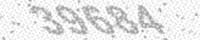Calculate Meaning in Urdu 1579

English Roman Urdu اردو
Calculate ginti karna گنتی کرنا

Calculate

make a mathematical calculation or computation

Synonyms

Account, Adjust, aim, Anticipate, Assume, Bet, cipher, compute, Consider, Count, count on, cypher, Depend, determine, direct, enumerate, Estimate, Figure, figure out, forecast, Guess, Judge, Look, make calculations, predict, reckon, reckon up, score, sum up, Suppose, tally, Total, Work

Browse Dictionary